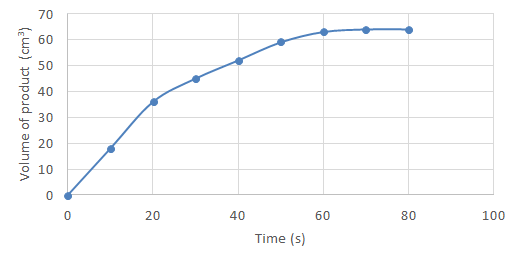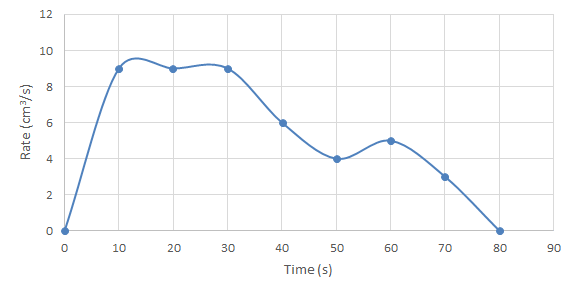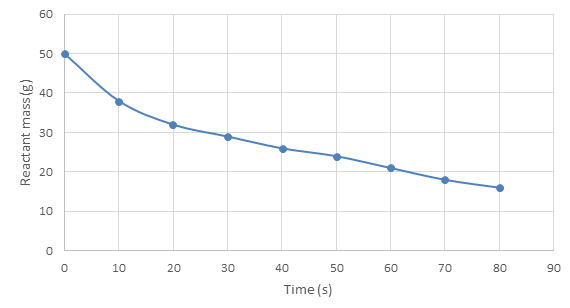# Reaction rate graphs

### Reaction rate graphs

#### Lessons

In this lesson, we will learn:

• How data from chemical reactions can be presented in graphs.
• How to interpret graphs showing data from chemical reactions.
Notes:

• After an investigation into the rate of a reaction, graphing the results to find the rate can be done in a number of ways, depending on what you measure and how you represent it.
• The most important thing to do when interpreting ANY graph is to read the axes – what are they measuring? What does a change in x or a change in y represent? Time should ALWAYS be displayed on the x-axis.
• This is very important when reading reaction rate graphs or graphing them yourself – you should choose how to display your data carefully.
• Reaction rate graphs often have very distinct curves or shapes which are strong evidence of a change in rate. However, these are very dependent on what is being displayed on the graph!
• On a graph of product formed over time:
• A flat curve (where gradient = 0) shows the reaction has stopped. Because your y-axis is measuring product formed, if it doesn't change, it is showing that more product has formed since the last data point, therefore the reaction has stopped and the reaction rate is zero. This normally happens after some time to show all the available produce has been made.
• The curve rising (y value increasing) shows more product is being formed – the reaction is still occurring.
• The steeper the gradient, the faster the reaction rate (greater $\frac{dy}{dx}$ )
• Product formed over time graphs do not show declines! Once a product is formed, it does not revert back to reactants. These graphs should not decline – if they do, there could be a mistake in your measurements.
• On a graph of change in reactant mass over time:
• A flat curve (where gradient = 0) shows the reaction has stopped. As above, this normally happens after some time to show the
• The general trend will be the y axis dropping – a declining curve, because reactant mass is going to be decreasing as products are formed.
• Again, the steeper the gradient, the faster the reaction rate (greater $\frac{dy}{dx}$ ) because the amount of reactant mass is decreasing more per unit time.
• In summary, these graphs are similar to the 'product formed over time' graphs except the curve generally drops instead of increases!
• On a graph of rate over time, the shape of the curve will have a different meaning:
• A flat curve means the reaction rate is constant. Unless y = 0, the y axis staying constant shows that the reaction is still occurring at the same rate it was when measured at the last data point.
• Any change in y is showing a change in rate – the reaction is speeding up or slowing down.
• The curve dropping to zero (the y-axis 0) shows the reaction has stopped.
• Introduction
Reaction rate graphs
a)
Product formed over time graphs.

b)
Reactant mass over time graphs.

c)
Rate over time graphs.

• 1.
Interpret graphs to describe chemical reactions by their reaction rate.
Interpret the graphs below to describe the chemical reaction in terms of its rate. Include the time when the reaction rate is highest, and when the reaction stops.
a)b)c)